### Mensuration Class 10th Mathematics Tamilnadu Board Solution

##### Question 1.The curved surface area of a right circular cylinder of radius 1 cm and height 1 cm is equal toA. πcm2B. 2πcm2C. 3π cm3D. 2 cm2Answer:Radius (R) = 1 cmHeight (H) = 1 cmCurved Surface Area = (2πRH) = 2π cm2Out of all the four Options,Correct Option: BQuestion 2.The total surface area of a solid right circular cylinder whose radius is half of its height h is equal toA.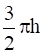sq. unitsB.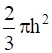sq. unitsC.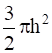sq. unitsD.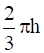sq. unitsAnswer:Total Surface Area = 2πrh + 2πr2where r is the radius and h is the heightAccording to the problem r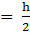Total surface area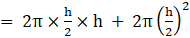⇒ Total surface area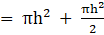⇒ Total surface area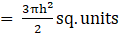Out of all the four Options,Correct Option : CQuestion 3.Base area of a right circular cylinder is 80 cm2. If its height is 5 cm, then the volume is equal toA. 400 cm3B. 16 cm3C. 200 cm3D.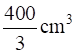Answer:Base Area (A) = 80 cm2Height (H) = 5 cmVolume = (A×H) = 400 cm3Out of all the four Options,Correct Option : AQuestion 4.If the total surface area a solid right circular cylinder is 200 πcm2and its radius is 5 cm, then the sum of its height and radius isA. 20 cmB. 25 cmC. 30 cmD. 15 cmAnswer:Total Surface Area = 2πrh + 2πr2where r is the radius and h is the heightTotal Surface Area = 200π cm2 (Given)Radius (r) = 5 cmEquating the Total Surface Area we can say2πrh + 2πr2 = 200π⇒ rh + r2 = 100⇒ r(r + h) = 100⇒ r + h = 20 cmOut of all the four Options,Correct Option : AQuestion 5.The curved surface area of a right circular cylinder whose radius is a units and height is b units, is equal toA. πa2b sq.cmB. 2πabC. 2π sq.cmD. 2 sq.cmAnswer:Radius (R) = a unitsHeight (H) = b unitsCurved Surface Area = (2πRH) = 2πab sq. unitsOut of all the four Options,Correct Option: BQuestion 6.Radius and height of a right circular cone and that of a right circular cylinder are respectively, equal. If the volume of the cylinder is 120 cm3, then the volume of the cone is equal toA. 1200 cm3B. 360 cm3C. 40 cm3D. 90 cm3Answer:Volume of Cylinder = 3 × Volume of ConeVolume of the cylinder = 120 cm3 (Given)⇒ Volume of Cone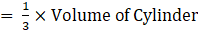⇒ Volume of Cone = 40 cm3Out of all the four Options,Correct Option : CQuestion 7.If the diameter and height of a right circular cone are 12 cm and 8 cm respectively, then the slant height isA. 10 cmB. 20 cmC. 30 cmD. 96 cmAnswer:Diameter = 12 cm.⇒ Radius (R) = 6 cm.Height (H) = 8 cmSlant height (l) = √(R2 + H2) = √(62 + 82)⇒ Slant height (l) = √100 = 10 cmOut of all the four Options,Correct Option : AQuestion 8.If the circumference at the base of a right circular cone and the slant height are 120 πcm and 10 cm respectively, then the curved surface area of the cone is equal toA. 1200πcm2B. 600πcm2C. 300πcm2D. 600 cm2Answer:Base Circumference = 120π cmLet radius be rBase Circumference = 2πr2πr = 120π⇒ r = 60 cmSlant Height (l) = 10 cmCurved Surface Area = π×r×lCurved Surface Area = 600π cm2Out of all the four Options,Correct Option :BQuestion 9.If the volume and the base area of a right circular cone are 48πcm3 and 12πcm2respectively, then the height of the cone is equal toA. 6 cmB. 8 cmC. 10 cmD. 12 cmAnswer:Volume of cone = 48π cm3Base Area = 12π cm2Volume of cone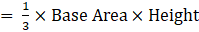Height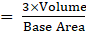⇒ Height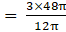⇒ Height = 12 cm.Out of all the four Options,Correct Option :DQuestion 10.If the height and the base area of a right circular cone are 5 cm and 48 sq. cm respectively, then the volume of the cone is equal toA. 240 cm3B. 120 cm3C. 80 cm3D. 480 cm3Answer:Base Area (A) = 48 cm2Height (H) = 5 cmVolume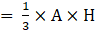⇒ Volume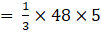⇒ Volume = 80 cm3Out of all the four Options,Correct Option : CQuestion 11.The ratios of the respective heights and the respective radii of two cylinders are 1:2 and 2:1 respectively. Then their respective volumes are in the ratioA. 4 : 1B. 1 : 4C. 2 : 1D. 1 : 2Answer:Let R1,R2 be the radius and H1,H2 be the height V1,V2 of the two cylindersVolume Of Cylinder = πR2HSo we can say,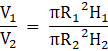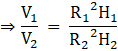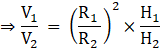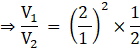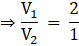Out of all the four Options,Correct Option : CQuestion 12.If the radius of a sphere is 2 cm, then the curved surface area of the sphere is equal toA. 8π cm2B. 16 cm2C. 12π cm2D. 16π cm2Answer:Radius of sphere (r) = 2 cmSurface Area of Sphere = 4πr2⇒ Surface Area of Sphere = 4π × 22⇒ Surface Area of Sphere = 16π cm2Out of all the four Options,Correct Option :DQuestion 13.The total surface area of a solid hemisphere of diameter 2 cm is equal toA. 12 cm2B. 12π cm2C. 4π cm2D. 3π cm2Answer:Diameter = 2 cmRadius (r) = 1 cmTotal surface Area = Curved surface Area + Base Area⇒ Total surface Area = 2πr2 + πr2 = 3πr2⇒ Total surface Area = 3π × 12 = 3π cm2Out of all the four Options,Correct Option : DQuestion 14.If the volume of a sphere is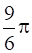cu. cm, then its radius isA.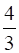cmB.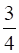cmC.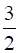cmD.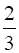cmAnswer:Let radius of sphere be r cmVolume of Sphere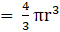Volume of Sphere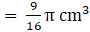So we can say that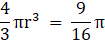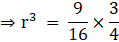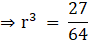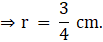Out of all the four Options,Correct Option : BQuestion 15.The surface areas of two spheres are in the ratio of 9 : 25. Then their volumes are in the ratioA. 81 : 625B. 729 : 15625C. 27 : 75D. 27 : 125Answer:Let R1,R2 be the radius of the two spheres and V1,V2 be the Volume of this two spheres, A1,A2 be the area of two spheresSurface Area of Sphere = 4πr2So we can say,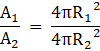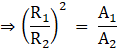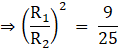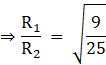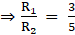…Equation (i)Volume of Sphere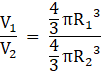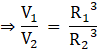Putting the values from Equation (i) we get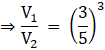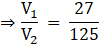Out of all the four Options,Correct Option : DQuestion 16.The total surface area of a solid hemisphere whose radius is a units, is equal toA. 2πa2sq. unitsB. 3πa2 sq. unitsC. 3πa sq. unitsD. 3a2 sq.unitsAnswer:Radius = a unitsTotal surface Area = Curved surface Area + Base Area⇒ Total surface Area = 2πa2 + πa2 = 3πa2 sq. unitsOut of all the four Options,Correct Option : BQuestion 17.If the surface area of a sphere is 100π cm2, then its radius is equal toA. 25 cmB. 100 cmC. 5 cmD. 10 cmAnswer:Let radius of sphere be r cmSurface Area of Sphere = 4πr2Surface Area of Sphere = 100π cm2So we can say,4πr2 = 100π⇒ r2 = 25⇒ r = 5 cmOut of all the four Options,Correct Option: CQuestion 18.If the surface area of a sphere is 36π cm2, then the volume of the sphere is equal toA. 12π cm3B. 36π cm3C. 72π cm3D. 108π cm3Answer:Let radius of sphere be r cmSurface Area of Sphere = 4πr2Surface Area of Sphere = 36π cm2So we can say,4πr2 = 36π⇒ r2 = 9⇒ r = 3 cmVolume of Sphere⇒ Volume of Sphere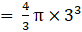⇒ Volume of Sphere = 4π × 32⇒ Volume of Sphere = 36π cm3Out of all the four Options,Correct Option : BQuestion 19.If the total surface area of a solid hemisphere is 12π cm2 then its curved surface area is equal toA. 6π cm2B. 24π cm2C. 36π cm2D. 8π cm2Answer:Total surface Area = Curved surface Area + Base Area⇒ Total surface Area = 2πr2 + πr2 = 3πr2Total surface area of a solid hemisphere = 12π cm2(Given)3πr2 = 12π⇒ r2 = 4⇒ r = 2cmCurved surface Area = 2πr2Curved surface Area = 2π×22Curved surface Area = 8π cm3Out of all the four Options,Correct Option : DQuestion 20.If the radius of a sphere is half of the radius of another sphere, then their respective volumes are in the ratioA. 1 : 8B. 2: 1C. 1 : 2D. 8 : 1Answer:Let R1,R2 be the radius of the two spheres and V1,V2 be the Volume of this two spheresVolume of Sphere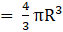R2 = 2R1So we can say,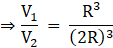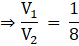Out of all the four Options,Correct Option :AQuestion 21.Curved surface area of solid sphere is 24 cm2. If the sphere is divided into two hemispheres, then the total surface area of one of the hemispheres isA. 12 cm2B. 8 cm2C. 16 cm2D. 18 cm2Answer:Curved Surface Area = 24 cm2Let radius be rCurved Surface Area of solid sphere = 4πr24πr2 = 24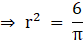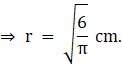Total surface Area of hemisphere = 2πr2 + πr2 = 3πr2Total surface Area of hemisphere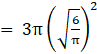Total surface Area of hemisphere = 18 cm2Out of all the four Options,Correct Option :DQuestion 22.Two right circular cones have equal radii. If their slant heights are in the ratio 4 : 3, then their respective curved surface areas are in the ratioA. 16 : 9B. 2 : 3C. 4 : 3D. 3 : 4Answer:Curved Surface Area = πRLwhere R is the radius and L is the slant heightLet L1,L2 be the slant heights of the two cone and A1,A2 be the area of this two conesSo we can say,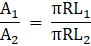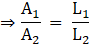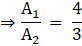Out of all the four Options,Correct Option : C

PDF FILE TO YOUR EMAIL IMMEDIATELY PURCHASE NOTES & PAPER SOLUTION. @ Rs. 50/- each (GST extra)

HINDI ENTIRE PAPER SOLUTION

MARATHI PAPER SOLUTION

SSC MATHS I PAPER SOLUTION

SSC MATHS II PAPER SOLUTION

SSC SCIENCE I PAPER SOLUTION

SSC SCIENCE II PAPER SOLUTION

SSC ENGLISH PAPER SOLUTION

SSC & HSC ENGLISH WRITING SKILL

HSC ACCOUNTS NOTES

HSC OCM NOTES

HSC ECONOMICS NOTES

HSC SECRETARIAL PRACTICE NOTES

2019 Board Paper Solution

HSC ENGLISH SET A 2019 21st February, 2019

HSC ENGLISH SET B 2019 21st February, 2019

HSC ENGLISH SET C 2019 21st February, 2019

HSC ENGLISH SET D 2019 21st February, 2019

SECRETARIAL PRACTICE (S.P) 2019 25th February, 2019

HSC XII PHYSICS 2019 25th February, 2019

CHEMISTRY XII HSC SOLUTION 27th, February, 2019

OCM PAPER SOLUTION 2019 27th, February, 2019

HSC MATHS PAPER SOLUTION COMMERCE, 2nd March, 2019

HSC MATHS PAPER SOLUTION SCIENCE 2nd, March, 2019

SSC ENGLISH STD 10 5TH MARCH, 2019.

HSC XII ACCOUNTS 2019 6th March, 2019

HSC XII BIOLOGY 2019 6TH March, 2019

HSC XII ECONOMICS 9Th March 2019

SSC Maths I March 2019 Solution 10th Standard11th, March, 2019

SSC MATHS II MARCH 2019 SOLUTION 10TH STD.13th March, 2019

SSC SCIENCE I MARCH 2019 SOLUTION 10TH STD. 15th March, 2019.

SSC SCIENCE II MARCH 2019 SOLUTION 10TH STD. 18th March, 2019.

SSC SOCIAL SCIENCE I MARCH 2019 SOLUTION20th March, 2019

SSC SOCIAL SCIENCE II MARCH 2019 SOLUTION, 22nd March, 2019

XII CBSE - BOARD - MARCH - 2019 ENGLISH - QP + SOLUTIONS, 2nd March, 2019

HSC Maharashtra Board Papers 2020

(Std 12th English Medium)

HSC ECONOMICS MARCH 2020

HSC OCM MARCH 2020

HSC ACCOUNTS MARCH 2020

HSC S.P. MARCH 2020

HSC ENGLISH MARCH 2020

HSC HINDI MARCH 2020

HSC MARATHI MARCH 2020

HSC MATHS MARCH 2020

SSC Maharashtra Board Papers 2020

(Std 10th English Medium)

English MARCH 2020

HindI MARCH 2020

Hindi (Composite) MARCH 2020

Marathi MARCH 2020

Mathematics (Paper 1) MARCH 2020

Mathematics (Paper 2) MARCH 2020

Sanskrit MARCH 2020

Sanskrit (Composite) MARCH 2020

Science (Paper 1) MARCH 2020

Science (Paper 2)

MUST REMEMBER THINGS on the day of Exam

Are you prepared? for English Grammar in Board Exam.

Paper Presentation In Board Exam

How to Score Good Marks in SSC Board Exams

Tips To Score More Than 90% Marks In 12th Board Exam

How to write English exams?

How to prepare for board exam when less time is left

How to memorise what you learn for board exam

No. 1 Simple Hack, you can try out, in preparing for Board Exam

How to Study for CBSE Class 10 Board Exams Subject Wise Tips?

JEE Main 2020 Registration Process – Exam Pattern & Important Dates

NEET UG 2020 Registration Process Exam Pattern & Important Dates

How can One Prepare for two Competitive Exams at the same time?

8 Proven Tips to Handle Anxiety before Exams!